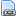Employee Benefits Survey
EBS# Technical Information about Standard Errors for Benefit Estimates

To assist users in ascertaining the reliability of National Compensation Survey (NCS) benefit estimates, standard errors of estimates are made available. Standard errors provide users a measure of the precision of an estimate to ensure that it is within an acceptable range for its intended purpose. Standard errors are available for the latest news release in HTML, PDF, and XLSX formats.

The estimates are derived from a sample survey and, thus, are subject to sampling errors. Sampling errors are differences that occur between the results computed from a sample of observations and those computed from all observations in a population. Estimates derived from different samples selected using the same sample design may differ from each other. (In this case, the population of an estimate is a worker characteristic, establishment characteristic, or geographic area.)

The standard error is a measure of the variation among these differing estimates. It can be used to measure the precision with which an estimate from a particular sample approximates the expected result of all possible samples. The standard errors can be used to define a range (confidence interval) around an estimate for a given confidence level. For instance, the 90 percent confidence level means that if all possible samples were selected and an estimate of a value and its sampling error were computed for each, then for approximately 90 percent of the samples, the intervals from 1.6 standard errors below the estimate to 1.6 standard errors above the estimate would include the "true" average value. For example, the 90 percent confidence interval for a benefit access rate estimate of 87 percent with a standard error of 0.7 percentage points would be 87 percent plus or minus 1.1 percentage points (1.6 standard errors multiplied by 0.7 percentage points) or 85.9 to 88.1 percent.

The chances are about 68 out of 100 percent that an estimate from the survey differs from the true population figure within one standard error. The chances are about 90 out of 100 percent that this difference would be within 1.6 standard errors. This means that in the example above, the chances are 90 out of 100 percent that the estimated benefit access rate is between 85.9 to 88.1 percent.

Comparative statements appearing in NCS benefit publications are statistically significant at the 90 percent level of confidence, unless otherwise indicated. This means that for differences cited, the estimated difference is greater than 1.6 times the standard error of the difference.

Employee Benefits in the United States, News Release (TXT) (PDF)